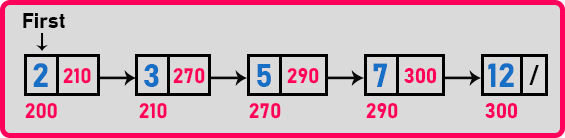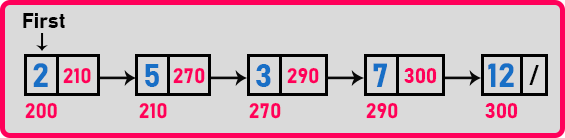# How to Check if a Linked List is Sorted

## How to Check if a Linked List is Sorted in C Language:

In this article, I am going to discuss How to Check if a Linked List is Sorted in C Programming Language with Examples. Please read our previous article, where we discussed How to Delete a Node from a Linked List using C Language with Examples.

##### Check if a Linked List is Sorted:

Here we will look at the procedure for finding whether a given linked list is sorted or not. In the below example, we have a linked list that is already sorted. All the elements are sorted and we have to check whether the linked list is sorted or not.Let us look at the procedure. The procedure is we should start from the first node. There is nothing previous to the first node, so let’s move to the next node. Now the current node value should be greater than the previous one. Here ‘3’ is greater than ‘2’. Then move to the next node which is ‘5’. ‘5’ is greater than the previous node which is ‘3’. So, in this way, we will check whether a linked list is sorted or not. The above LinkedList is already sorted. Suppose we have one unsorted linked list as follows:Let’s iterate again. We should start with the first node which is node ‘2’. As there is no node previous to node ‘2’ so move to the next node. Node ‘5’ is greater than node ‘2’ then move to the next node. Now node ‘3’ is not greater than node ‘5’. So, this is not a sorted list. So just check the condition that we are getting any value that is smaller than the previous value, if so, it is not sorted. If not, you have reached the end of the list you have checked all the nodes then the list will be sorted list. So let us see a procedure for this one.We need the value of the current node and the value of the previous node. So, we will take a variable let’s say ‘x’, and let it be our minimum integer i.e. -32768. Now take a pointer ‘p’ on the first node. Then check if the data of the first node is greater than ‘x’. So ‘2’ is greater than ‘x’ or -32768. So, modify ‘x’ to ‘2’. Then move ‘p’ to the next node.Here ‘p’ is on the ‘5’ node. Again check, if (x < p->data), yes, so change ‘x’ to ‘5’. Then move ‘p’ to the next node.Now, ‘p’ is pointing on the ‘3’ node. So, check if (x < p->data), no. So here we got a value that is not in increasing order. Here we have to stop and say the linked list is not sorted. Suppose all the values of the above LinkedList are sorted then the ‘p’ pointer will trace the whole linked list until ‘p’ points to null and return true as the linked list is sorted. Now let us write pseudocode.

##### Check if a Linked List is Sorted Pseudo Code:

int x = -32768;
Node *p = first;
while(p != NULL){
if(p->data < x)
return false;
x = p->data;
p = p->next;
}
return true;

In the above code, we are checking for failure conditions in if-block that is if p’s data is smaller than ‘x’ then the list is not sorted. Let us trace a linked list with this code.

##### Example-
###### Step1-First, the while loop condition will check. Here ‘p’ is not null then while loop will execute. Next ‘if(p->data < x)’, no so statements of ‘if’ block will not execute. Then ‘x=p->data’ so ‘x’ is ‘4’ now and ‘p=p->next’.

###### Step2-Here again, the while loop condition will check. ‘p’ is not pointing on null so it will execute statements inside the while loop. Next ‘if(p->data < x)’, no so ‘if’ block will not execute. Then ‘x=p->data’ so ‘x’ is ‘5’ now and ‘p=p->next’.

###### Step3-The same steps will be followed here. Here the value of ‘x’ is ‘7’ and ‘p’ will point to the next node.

###### Step4-Hereafter entering the while loop, the condition for the ‘if’ block will be checked that is ‘if (p->data > x)’, this will be true as ‘3 < 7’ so it will return false that is linked list is not sorted. Now let us look at the complete program for checking whether the linked list is sorted or not.

##### Program for Checking if a Linked List is Sorted using C Language:
```#include <stdio.h>
#include <stdlib.h>

struct Node
{
int data;
struct Node *next;
}   *first = NULL;

void Create(int A[], int n)
{
int i;
struct Node *t, *last;
first = (struct Node *) malloc(sizeof(struct Node));
first->data = A;
first->next = NULL;
last = first;

for (i = 1; i < n; i++)
{
t = (struct Node *) malloc(sizeof(struct Node));
t->data = A[i];
t->next = NULL;
last->next = t;
last = t;
}
}

void Display(struct Node *p)
{
while (p != NULL)
{
printf ("%d ", p->data);
p = p->next;
}
}

int IsSorted(struct Node *p)
{
int x = -65536;

while (p != NULL)
{
if (p->data < x)
return 0;
x = p->data;
p = p->next;
}
return 1;
}

int main()
{
int A[] = { 4, 5, 7, 11, 12 };
Create(A, 5);
Display (first);
if(IsSorted(first))